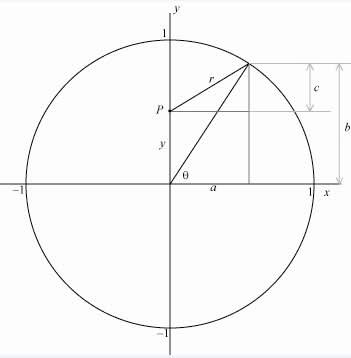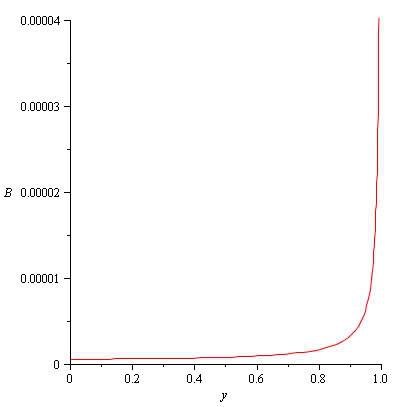# Magnetic Field in Plane of Loop

pidoctor314
1.
I have a tricky problem here. Using biot-savart's law, I need to find an expression for the magnetic field inside a loop anywhere on the plane of the loop. I am not expected to solve the integral, only set it up.

2. Biot-Savart's law is...
$$d\textbf{B} = \frac{\mu_0}{4\pi} \frac{Id\textbf{l} \times \textbf{\hat{r}}}{r^2}$$

3. I can solve for along the axis, but I am at a loss for how to do this problem. Any tips for solving would help! Thank you, this is an amazing site.

What your doing in the Biot-Savart Law is integrating along a path $$\textbf{l}$$. Also, $$\textbf{r}$$ is the displacement of some point from $$d\textbf{l}$$, so it isn't constant. In this case, your path is a loop of wire.

It might help to re-write the Law like this:

$$\frac{d\textbf{B}}{dt} = \frac{\mu_0}{4\pi} \frac{I\frac{d\textbf{l}}{dt} \times \textbf{r}}{r^3}$$

very difficult to solve that integration, at least with fundamental integration formulas.

higher mathematics will be required, if it can be done.

Are you sure you need to solve it everywhere on the plane? Looks a tad hard to me tbh...
Maybe they mean only on the x and y axis for example? Or on the entire line through the center of the loop?

pidoctor314
Yes, this is an 'honors' physics course, so they don't really pull any punches. I am sure it is anywhere on the plane on the loop.
for the quation that Foxjwill wrote down...that defenitely seems more useful than the orignial form, but why is there a dt (as I would imagine the final answer be with respect to position)

but why is there a dt (as I would imagine the final answer be with respect to position)

I put the dt in just to show that you're taking derivatives--it's really just a "ghost" variable because, essentially, it just cancels out.

The dt is there because the formula is given for $\frac{dB}{dt}$, not just $dB$.

If you really have to find the magnetic field on the entire plane, your best bet is probably to find some kind of position function or something...

Maybe you can use polar coordinates, where the angle and radial distance denote a point inside the loop (this way, you don't have to worry about the shape being a loop, which could get troublesome with cartesian coordinates).

For instance, you can define a point on the plane inside the loop with:
$$P : (\theta, r)$$
where $r$ is the distance of a line from the origin (in the center of your loop) and some point on the loop, and $\theta$ is the angle between the positive x-axis and this line.
Note that you can then quickly say $r < R$ where R is the radius of the loop.

Then, to use biot-savart, it gets a bit more tricky. You have to find some kind of relation for the distance from the point P to all positions on the loop (this is the $r$ you use in biot-savart's law).

To be honest I don't know if this is a good way to do this (I never did this before) and I expect someone to come up with a way easier and better solution soon... But if not, I might even try it myself if I find the time, quite interesting.

Wait... Maybe I already found a way to do it much easier :p

Imagine the loop lying in the xy-plane. If you can find the magnetic field everywhere on the x-axis for example, it will be exactly the same everywhere on the y-axis, since the loop is symmetrical.

So if you know what the field is on the x or y axis, you know the field everywhere in the plane of the loop.

Ok, I did some work on this.

I don't think I did this correctly... I probably did some thing(s) wrong... So if someone can please correct me that would be great! I hope I'm not too far off though...

Consider the loop lying in the xy-plane as the following image shows:To simplify the expressions a bit I chose the radius of the loop to be 1.

The point P is the point on which I'm going to calculate the magnetic field. This point lies on the y-axis, a distance $y$ from the origin.

The distance $r$ from point P to any point on the loop can be calculated as follows:

Because of the right triangle:
$$r^2 = a^2 + c^2 = a^2 + (b-y)^2$$

$a$ follows directly from the angle $\theta$, and the some goes for $b$:
$$a = \cos \theta$$
$$b = \sin \theta$$

So we find:
$$r^2 = (\cos \theta)^2 + (\sin \theta - y)^2$$

Now, the length of a small piece of the loop $dl$ is simply the radius of the loop multiplied by a small angle $d \theta$. Since the radius is 1:
$$dl = d \theta$$

Now finally for biot-savart's law we get:
$$dB = \frac{\mu_0 I}{4 \pi} \frac{d \theta}{(\cos \theta)^2 + (\sin \theta - y)^2}$$

$$B = \frac{\mu_0 I}{4 \pi} \int_0^{2 \pi} \frac{d \theta}{(\cos \theta)^2 + (\sin \theta - y)^2}$$

I hope this is somewhat correct or only a bit in the right direction... I have little hope though, since when I tried to compute the integral using Maple I got something with complex logarithms...

However, I did try to plot the function for y from 0 to 1 (I = 1 A) and got the following graph:The field goes to infinity for y = 1 which is what I would expect (being inside the wire). I have no idea however if the general shape is correct at all...
EDIT
However, for y = 0, I get the same result as the usual 'field in the center of the loop' which is:
$$B = \frac{\mu_0 I}{2R} = \frac{1}{2} \mu_0$$ (center of loop with I = 1A and R = 1m)

Hope this helps.

Last edited:
pidoctor314
wow, thank you to any the replies
Nick89, your reply was very clear and easy to understand. I will recheck that, but it appears correct

what direction would the field be in? do all the directions that aren't point towards Z necessarily cancel or do u have to consider them seperatley for each small piece of wire.

Directly from Biot-Savart's law:
$$\vec{dB} = \frac{\mu_0}{4 \pi} \frac{ \vec{dl} \times \vec{e_r} }{r^2}$$
(where $\vec{e_r}$ is the direction of $r$)

If you look at any point on the loop for dl, and any point somewhere inside the plane of the loop for the point P (form which you calculate r), you will see that everywhere inside the plane of the loop, the magnetic field will be directed perpendicular on the plane (wether 'into the page' or 'out of the page' depends on the direction of the current ofcourse)